# Productive Rage

Dan's techie ramblings

## (Approximately) correcting perspective with C# (fixing a blurry presentation video - part two)

### TL;DR

I have a video of a presentation where the camera keeps losing focus such that the slides are unreadable. I have the original slide deck and I want to fix this.

Step one was identifying the area in each frame that it seemed likely was where the slides were being projected, now step two is to correct the perspective of the projection back into a rectangle to make it easier to perform comparisons against the original slide deck images and try to determine which slide was being projected.

(An experimental TL;DR approach: See this small scale .NET Fiddle demonstration of what I'll be discussing)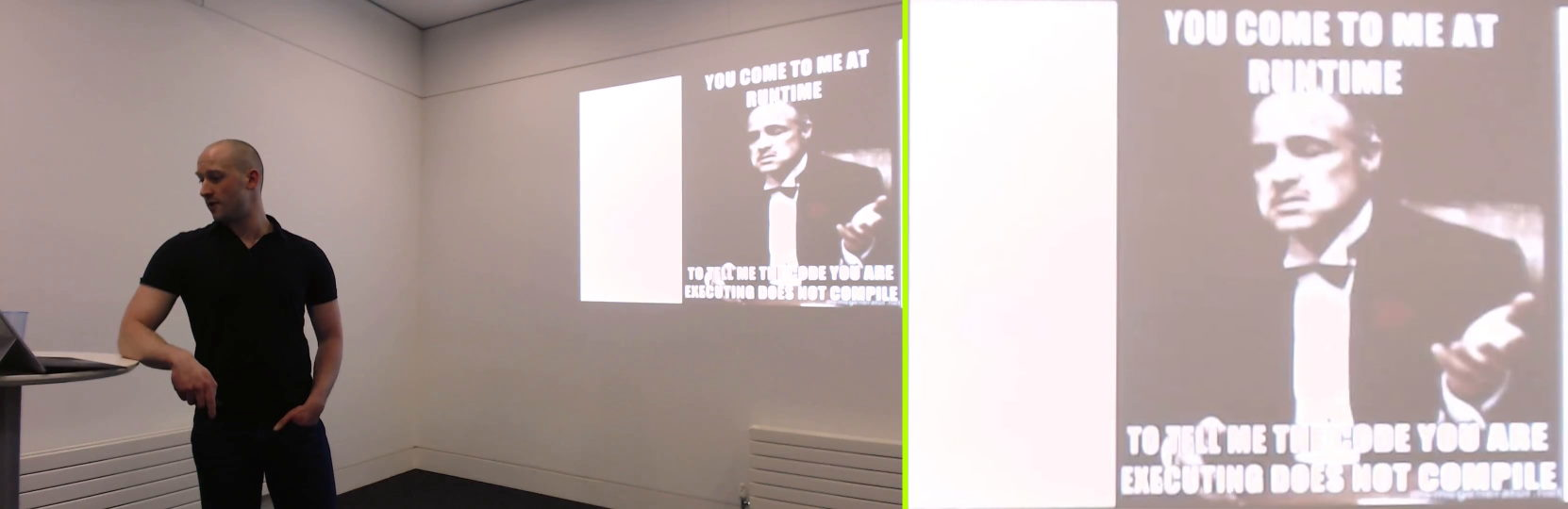### The basic approach

An overview of the processing to do this looks as follows:

1. Load the original slide image into a `Bitmap`
2. Using the projected-slide-area region calculated in step one..
1. Take the line from the top left of the region to the top right
2. Take the line from the bottom left of the region to the bottom right (note that this line may be a little longer or shorter than the first line)
3. Create vertical slices of the image by stepping through the first line (the one across the top), connecting each pixel to a pixel on the bottom line
3. These vertical slices will not all be the same height and so they'll need to be adjusted to a consistent size (the further from the camera that a vertical slice of the projection is, the smaller it will be)
4. The height-adjusted vertical slices are then combined into a single rectangle, which will result in an approximation of a perspective-corrected version of the projection of the slide

Note: The reason that this process is only going to be an approximation is due to the way that the height of the output image will be determined -

1. For my purposes, it will be fine to use the largest of the top-left-to-bottom-left length (ie. the left-hand edge of the projection) and the top-right-to-bottom-right length (the right-hand edge of the projected) but this will always result in an output whose aspect ratio is stretched vertically slightly because the largest of those two lengths will be "magnified" somewhat due to the perspective effect
2. What might seem like an obvious improvement would be to take an average of the left-hand-edge-height and the right-hand-edge-height but I decided not to do this because I would be losing some fidelity from the vertical slices that would be shrunken down to match this average and because this would still be an approximation as..
3. The correct way to determine the appropriate aspect ratio for the perspective-corrected image is to use some clever maths to try to determine that angle of the wall that the projection is on (look up perspective correction and vanishing points if you're really curious!) and to use that to decide what ratio of the left-hand-edge-height and the right-hand-edge-height to use
• (The reason that the take-an-average approach is still an approximation is that perspective makes the larger edge grow more quickly than the smaller edge shrinks, so this calculation would still skew towards a vertically-stretched image)

### Slice & dice!

So if we follow the plan above then we'll generate a list of vertical slices a bit like this: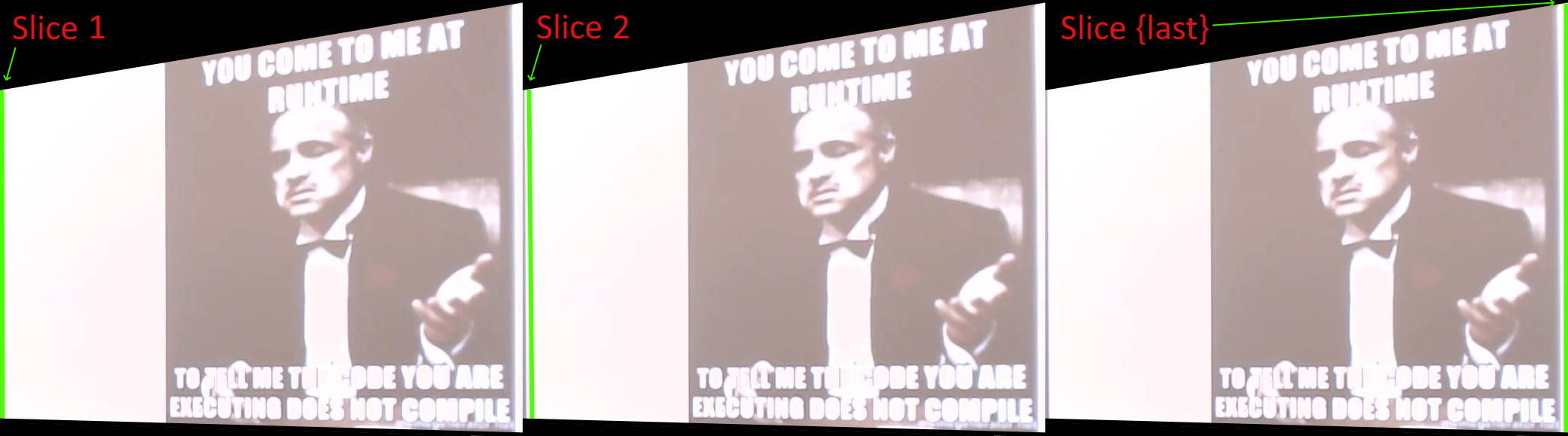.. which, when combined would look like this: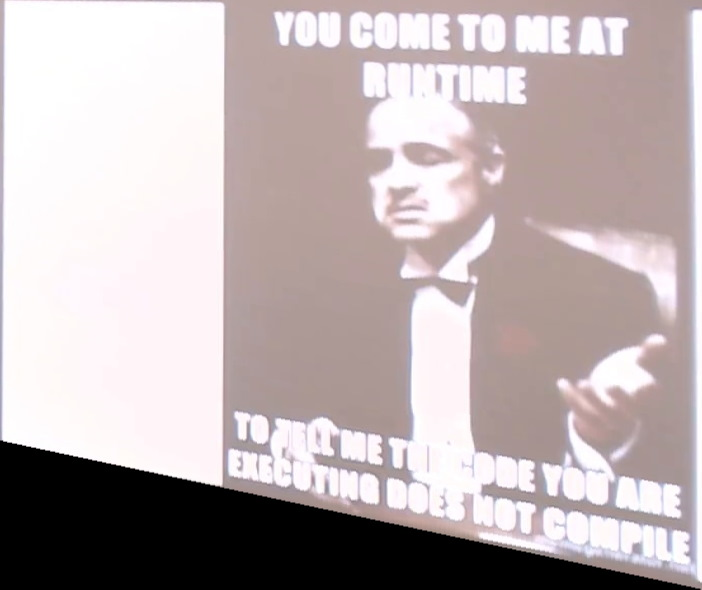This is very similar to the original projection except that:

• The top edge is now across the top of the rectangular area
• The bottom left corner is aligned with the left-hand side of the image
• The bottom right corner is aligned with the right-hand side of the image

We're not done yet but this has brought things much closer!

In fact, all that is needed is to stretch those vertical slices so that they are all the same length and; ta-da!### Implementation for slicing and stretching

So, from previous analysis, I know that the bounding area for the projection of the slide in the frames of my video is:

``````topLeft: (1224, 197)
topRight: (1915, 72)

bottomLeft: (1229, 638)
bottomRight: (1915, 662)
``````

Since I'm going to walk along the top edge and create vertical slices from that, I'm going to need the length of that edge - which is easy enough with some Pythagoras:

``````private static int LengthOfLine((PointF From, PointF To) line)
{
var deltaX = line.To.X - line.From.X;
var deltaY = line.To.Y - line.From.Y;
return (int)Math.Round(Math.Sqrt((deltaX * deltaX) + (deltaY * deltaY)));
}
``````

So although it's only 691px horizontally from the top left to the top right (1915 - 1224), the actual length of that edge is 702px (because it's not a line that angles up slightly rather than being a flat horizontal one).

This edge length determines how many vertical slices that we'll take and we'll get them by looping across this top edge, working out where the corresponding point on the bottom edge should be and joining them together into a line; one vertical slice. Each time that the loop increments, the current point on the top edge is going to move slightly to the right and even more slightly upwards while each corresponding point on the bottom edge will also move slightly to the right but it will move slightly down as the projection on the wall gets closer and closer to the camera.

One way to get all of these vertical slice lines is a method such as the following:

``````private sealed record ProjectionDetails(
Size ProjectionSize,
IEnumerable<((PointF From, PointF To) Line, int Index)> VerticalSlices
);

private static ProjectionDetails GetProjectionDetails(
Point topLeft,
Point topRight,
Point bottomRight,
Point bottomLeft)
{
var topEdge = (From: topLeft, To: topRight);
var bottomEdge = (From: bottomLeft, To: bottomRight);
var lengthOfEdgeToStartFrom = LengthOfLine(topEdge);
var dimensions = new Size(
width: lengthOfEdgeToStartFrom,
height: Math.Max(
LengthOfLine((topLeft, bottomLeft)),
LengthOfLine((topRight, bottomRight))
)
);
return new ProjectionDetails(dimensions, GetVerticalSlices());

IEnumerable<((PointF From, PointF To) Line, int Index)> GetVerticalSlices() =>
Enumerable
.Range(0, lengthOfEdgeToStartFrom)
.Select(i =>
{
var fractionOfProgressAlongPrimaryEdge = (float)i / lengthOfEdgeToStartFrom;
return (
Line: (
GetPointAlongLine(topEdge, fractionOfProgressAlongPrimaryEdge),
GetPointAlongLine(bottomEdge, fractionOfProgressAlongPrimaryEdge)
),
Index: i
);
});
}
``````

This returns the dimensions of the final perspective-corrected projection (which is as wide as the top edge is long and which is as high as the greater of the left-hand edge's length and the right-hand edge's length) as well as an `IEnumerable` of the start and end points for each slice that we'll be taking.

The dimensions are going to allow us to create a bitmap that we'll paste the slices into when we're ready - but, before that, we need to determine pixel values for every point on every vertical slice. As the horizontal distance across the top edge is 691px and the vertical distance is 125px but its actual length is 702px, each time we move one along in that 702px loop the starting point for the vertical slice will move (691 / 702) = 0.98px across and (125 / 702) = 0.18px up. So almost all of these vertical slices are going to have start and end points that are not whole pixel values - and the same applies to each point on that vertical slice. This means that we're going to have to take average colour values for when we're dealing with fractional pixel locations.

For example, if we're at the point (1309.5, 381.5) and the colours at (1309, 381), (1310, 381), (1309, 382), (1310, 382) are all white then the averaging is really easy - the "averaged" colour is white! If we're at the point (1446.5, 431.5) and the colours at (1446, 431), (1447, 431), (1446, 432), (1447, 432) are #BCA6A9, #B1989C, #BCA6A9, #B1989C then it's also not too complicated - because (1446.5, 431.5) is at the precise midpoint between all four points then we can take a really simple average by adding all four R values together, all four G values together, all four B values together and diving them by 4 to get a combined result. It gets a little more complicated where it's not 0.5 of a pixel and it's slightly more to the left or to the right and/or to the top or to the bottom - eg. (1446.1, 431.9) would get more of its averaged colour from the pixels on the left than on the right (as 1446.1 is only just past 1446) while it would get more of its averaged colour from the pixels on the bottom than the top (as 431.9 is practically ay 432). On the other hand, on the rare occasion where it is a precise location (with no fractional pixel values), such as (1826, 258), then it's the absolute simplest case because no averaging is required!

``````private static Color GetAverageColour(Bitmap image, PointF point)
{
var (integralX, fractionalX) = GetIntegralAndFractional(point.X);
var x0 = integralX;
var x1 = Math.Min(integralX + 1, image.Width);

var (integralY, fractionalY) = GetIntegralAndFractional(point.Y);
var y0 = integralY;
var y1 = Math.Min(integralY + 1, image.Height);

var (topColour0, topColour1) = GetColours(new Point(x0, y0), new Point(x1, y0));
var (bottomColour0, bottomColour1) = GetColours(new Point(x0, y1), new Point(x1, y1));

return CombineColours(
CombineColours(topColour0, topColour1, fractionalX),
CombineColours(bottomColour0, bottomColour1, fractionalX),
fractionalY
);

(Color c0, Color c1) GetColours(Point p0, Point p1)
{
var c0 = image.GetPixel(p0.X, p0.Y);
var c1 = (p0 == p1) ? c0 : image.GetPixel(p1.X, p1.Y);
return (c0, c1);
}

static (int Integral, float Fractional) GetIntegralAndFractional(float value)
{
var integral = (int)Math.Truncate(value);
var fractional = value - integral;
return (integral, fractional);
}

static Color CombineColours(Color x, Color y, float proportionOfSecondColour)
{
if ((proportionOfSecondColour == 0) || (x == y))
return x;

if (proportionOfSecondColour == 1)
return y;

return Color.FromArgb(
red: CombineComponent(x.R, y.R),
green: CombineComponent(x.G, y.G),
blue: CombineComponent(x.B, y.B),
alpha: CombineComponent(x.A, y.A)
);

int CombineComponent(int x, int y) =>
Math.Min(
(int)Math.Round((x * (1 - proportionOfSecondColour)) + (y * proportionOfSecondColour)),
255
);
}
}
``````

This gives us the capability to split the wonky projection into vertical slices, to loop over each slice and to walk down each slice and get a list of pixel values for each point down that slice. The final piece of the puzzle is that we then need to resize each vertical slice so that they all match the projection height returned from the `GetProjectionDetails` method earlier. Handily, the .NET `Bitmap` drawing code has `DrawImage` functionality that can resize content, so we can:

1. Create a `Bitmap` whose dimensions are those returned from `GetProjectionDetails`
2. Loop over each vertical slice (which is an `IEnumerable` also returned from `GetProjectionDetails`)
3. Create a bitmap just for that slice - that is 1px wide and only as tall as the current vertical slice is long
4. Use `DrawImage` to paste that slice's bitmap onto the full-size projection `Bitmap`

In code:

``````private static void RenderSlice(
Bitmap projectionBitmap,
IEnumerable<Color> pixelsOnLine,
int index)
{
var pixelsOnLineArray = pixelsOnLine.ToArray();

using var slice = new Bitmap(1, pixelsOnLineArray.Length);
for (var j = 0; j < pixelsOnLineArray.Length; j++)
slice.SetPixel(0, j, pixelsOnLineArray[j]);

using var g = Graphics.FromImage(projectionBitmap);
g.DrawImage(
slice,
srcRect: new Rectangle(0, 0, slice.Width, slice.Height),
destRect: new Rectangle(index, 0, 1, projectionBitmap.Height),
srcUnit: GraphicsUnit.Pixel
);
}
``````

### Pulling it all together

If we combine all of this logic together then we end up with a fairly straightforward static class that does all the work - takes a `Bitmap` that is a frame from a video where there is a section that should be extracted and then "perspective-corrected", takes the four points that describe that region and then returns a new `Bitmap` that is the extracted content in a lovely rectangle!

``````/// <summary>
/// This uses a simple algorithm to try to undo the distortion of a rectangle in an image
/// due to perspective - it takes the content of the rectangle and stretches it into a
/// rectangle. This is only a simple approximation and does not guarantee accuracy (in
/// fact, it will result in an image that is slightly vertically stretched such that its
/// aspect ratio will not match the original content and a more thorough approach would
/// be necessary if this is too great an approximation)
/// </summary>
internal static class SimplePerspectiveCorrection
{
public static Bitmap ExtractAndPerspectiveCorrect(
Bitmap image,
Point topLeft,
Point topRight,
Point bottomRight,
Point bottomLeft)
{
var (projectionSize, verticalSlices) =
GetProjectionDetails(topLeft, topRight, bottomRight, bottomLeft);

var projection = new Bitmap(projectionSize.Width, projectionSize.Height);
foreach (var (lineToTrace, index) in verticalSlices)
{
var lengthOfLineToTrace = LengthOfLine(lineToTrace);

var pixelsOnLine = Enumerable
.Range(0, lengthOfLineToTrace)
.Select(j =>
{
var fractionOfProgressAlongLineToTrace = (float)j / lengthOfLineToTrace;
var point = GetPointAlongLine(lineToTrace, fractionOfProgressAlongLineToTrace);
return GetAverageColour(image, point);
});

RenderSlice(projection, pixelsOnLine, index);
}
return projection;

static Color GetAverageColour(Bitmap image, PointF point)
{
var (integralX, fractionalX) = GetIntegralAndFractional(point.X);
var x0 = integralX;
var x1 = Math.Min(integralX + 1, image.Width);

var (integralY, fractionalY) = GetIntegralAndFractional(point.Y);
var y0 = integralY;
var y1 = Math.Min(integralY + 1, image.Height);

var (topColour0, topColour1) = GetColours(new Point(x0, y0), new Point(x1, y0));
var (bottomColour0, bottomColour1) = GetColours(new Point(x0, y1), new Point(x1, y1));

return CombineColours(
CombineColours(topColour0, topColour1, fractionalX),
CombineColours(bottomColour0, bottomColour1, fractionalX),
fractionalY
);

(Color c0, Color c1) GetColours(Point p0, Point p1)
{
var c0 = image.GetPixel(p0.X, p0.Y);
var c1 = (p0 == p1) ? c0 : image.GetPixel(p1.X, p1.Y);
return (c0, c1);
}

static (int Integral, float Fractional) GetIntegralAndFractional(float value)
{
var integral = (int)Math.Truncate(value);
var fractional = value - integral;
return (integral, fractional);
}

static Color CombineColours(Color x, Color y, float proportionOfSecondColour)
{
if ((proportionOfSecondColour == 0) || (x == y))
return x;

if (proportionOfSecondColour == 1)
return y;

return Color.FromArgb(
red: CombineComponent(x.R, y.R),
green: CombineComponent(x.G, y.G),
blue: CombineComponent(x.B, y.B),
alpha: CombineComponent(x.A, y.A)
);

int CombineComponent(int x, int y) =>
Math.Min(
(int)Math.Round(
(x * (1 - proportionOfSecondColour)) +
(y * proportionOfSecondColour)
),
255
);
}
}
}

private sealed record ProjectionDetails(
Size ProjectionSize,
IEnumerable<((PointF From, PointF To) Line, int Index)> VerticalSlices
);

private static ProjectionDetails GetProjectionDetails(
Point topLeft,
Point topRight,
Point bottomRight,
Point bottomLeft)
{
var topEdge = (From: topLeft, To: topRight);
var bottomEdge = (From: bottomLeft, To: bottomRight);
var lengthOfEdgeToStartFrom = LengthOfLine(topEdge);
var dimensions = new Size(
width: lengthOfEdgeToStartFrom,
height: Math.Max(
LengthOfLine((topLeft, bottomLeft)),
LengthOfLine((topRight, bottomRight))
)
);
return new ProjectionDetails(dimensions, GetVerticalSlices());

IEnumerable<((PointF From, PointF To) Line, int Index)> GetVerticalSlices() =>
Enumerable
.Range(0, lengthOfEdgeToStartFrom)
.Select(i =>
{
var fractionOfProgressAlongPrimaryEdge = (float)i / lengthOfEdgeToStartFrom;
return (
Line: (
GetPointAlongLine(topEdge, fractionOfProgressAlongPrimaryEdge),
GetPointAlongLine(bottomEdge, fractionOfProgressAlongPrimaryEdge)
),
Index: i
);
});
}

private static PointF GetPointAlongLine((PointF From, PointF To) line, float fraction)
{
var deltaX = line.To.X - line.From.X;
var deltaY = line.To.Y - line.From.Y;
return new PointF(
(deltaX * fraction) + line.From.X,
(deltaY * fraction) + line.From.Y
);
}

private static int LengthOfLine((PointF From, PointF To) line)
{
var deltaX = line.To.X - line.From.X;
var deltaY = line.To.Y - line.From.Y;
return (int)Math.Round(Math.Sqrt((deltaX * deltaX) + (deltaY * deltaY)));
}

private static void RenderSlice(
Bitmap projectionBitmap,
IEnumerable<Color> pixelsOnLine,
int index)
{
var pixelsOnLineArray = pixelsOnLine.ToArray();

using var slice = new Bitmap(1, pixelsOnLineArray.Length);
for (var j = 0; j < pixelsOnLineArray.Length; j++)
slice.SetPixel(0, j, pixelsOnLineArray[j]);

using var g = Graphics.FromImage(projectionBitmap);
g.DrawImage(
slice,
srcRect: new Rectangle(0, 0, slice.Width, slice.Height),
destRect: new Rectangle(index, 0, 1, projectionBitmap.Height),
srcUnit: GraphicsUnit.Pixel
);
}
}
``````

### Coming next

So step one was to take frames from a video and to work out what the bounds were of the area where slides were being projected (and to filter out any intro and outro frames), step two has been to be able to take the bounded area from any slide and project it back into a rectangle to make it easier to match against the original slide images.. step three will be to use these projections to try to guess what slide is being displayed on what frame!

The frame that I've been using as an example throughout this post probably looks like a fairly easy case - big blocks of white or black and not actually too out of focus.. but some of the frames look like this and that's a whole other kettle of fish!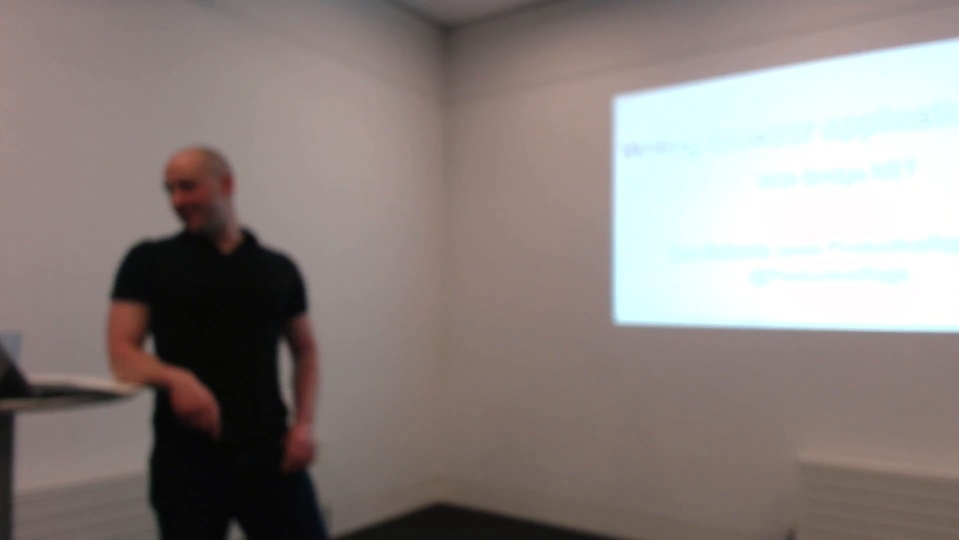Posted at 19:02

## Finding the brightest area in an image with C# (fixing a blurry presentation video - part one)

### TL;DR

I have a video of a presentation where the camera keeps losing focus such that the slides are unreadable. I have the original slide deck and I want to fix this.

The first step is analysing the individual frames of the video to find a common "most illuminated area" so that I can work out where the slide content was being projected, and that is what is described in this post.

(An experimental TL;DR approach: See this small scale .NET Fiddle demonstration of what I'll be discussing)### The basic approach

An overview of the processing to do this looks as follows:

1. Load the image into a `Bitmap`
2. Convert the image to greyscale
3. Identify the lightest and darkest values in the greyscale range
4. Calculate a 2/3 threshold from that range and create a mask of the image where anything below that value is zero and anything equal to or greater is one
• eg. If the darkest value was 10 and the lightest was 220 then the difference is 220 - 10 = 210 and the cutoff point would be 2/3 of this range on top of the minimum, so the threshold value would equal ((2/3) * range) + minimum = ((2/3) * 210) + 10 = 140 + 10 = 150
5. Find the largest bounded area within this mask (if there is one) and presume that that's the projection of the slide in the darkened room!

Before looking at code to do that, I'm going to toss in a few other complications that arise from having to process a lot of frames from throughout the video, rather than just one..

Firstly, the camera loses focus at different points in the video and to different extents and so some frames are blurrier than others. Following the steps above, the blurrier frames are likely to report a larger projection area for the slides. I would really like to identify a common projection area that is reasonable to use across all frames because this will make later processing (where I try to work out what slide is currently being shown in the frame) easier.

Secondly, this video has intro and outro animations and it would be nice if I was able to write code that worked out when they stopped and started.

### The implementation for a single image

To do this work, I'm going to introduce a variation of my old friend the `DataRectangle` (from "How are barcodes read?" and "Face or no face") -

``````public static class DataRectangle
{
public static DataRectangle<T> For<T>(T[,] values) => new DataRectangle<T>(values);
}

public sealed class DataRectangle<T>
{
private readonly T[,] _protectedValues;
public DataRectangle(T[,] values) : this(values, isolationCopyMayBeBypassed: false) { }
private DataRectangle(T[,] values, bool isolationCopyMayBeBypassed)
{
if ((values.GetLowerBound(0) != 0) || (values.GetLowerBound(1) != 0))
throw new ArgumentException("Both dimensions must have lower bound zero");
var arrayWidth = values.GetUpperBound(0) + 1;
var arrayHeight = values.GetUpperBound(1) + 1;
if ((arrayWidth == 0) || (arrayHeight == 0))
throw new ArgumentException("zero element arrays are not supported");

Width = arrayWidth;
Height = arrayHeight;

if (isolationCopyMayBeBypassed)
_protectedValues = values;
else
{
_protectedValues = new T[Width, Height];
Array.Copy(values, _protectedValues, Width * Height);
}
}

public int Width { get; }

public int Height { get; }

public T this[int x, int y]
{
get
{
if ((x < 0) || (x >= Width))
throw new ArgumentOutOfRangeException(nameof(x));
if ((y < 0) || (y >= Height))
throw new ArgumentOutOfRangeException(nameof(y));
return _protectedValues[x, y];
}
}

public IEnumerable<(Point Point, T Value)> Enumerate()
{
for (var x = 0; x < Width; x++)
{
for (var y = 0; y < Height; y++)
{
var value = _protectedValues[x, y];
var point = new Point(x, y);
yield return (point, value);
}
}
}

public DataRectangle<TResult> Transform<TResult>(Func<T, TResult> transformer)
{
var transformed = new TResult[Width, Height];
for (var x = 0; x < Width; x++)
{
for (var y = 0; y < Height; y++)
transformed[x, y] = transformer(_protectedValues[x, y]);
}
return new DataRectangle<TResult>(transformed, isolationCopyMayBeBypassed: true);
}
}
``````

For working with `DataRectangle` instances that contain `double` values (as we will be here), I've got a couple of convenient extension methods:

``````public static class DataRectangleOfDoubleExtensions
{
public static (double Min, double Max) GetMinAndMax(this DataRectangle<double> source) =>
source
.Enumerate()
.Select(pointAndValue => pointAndValue.Value)
.Aggregate(
seed: (Min: double.MaxValue, Max: double.MinValue),
func: (acc, value) => (Math.Min(value, acc.Min), Math.Max(value, acc.Max))
);

public static DataRectangle<bool> Mask(this DataRectangle<double> values, double threshold) =>
values.Transform(value => value >= threshold);
}
``````

And for working with `Bitmap` instances, I've got some extension methods for those as well:

``````public static class BitmapExtensions
{
public static Bitmap CopyAndResize(this Bitmap image, int resizeLargestSideTo)
{
var (width, height) = (image.Width > image.Height)
? (resizeLargestSideTo, (int)((double)image.Height / image.Width * resizeLargestSideTo))
: ((int)((double)image.Width / image.Height * resizeLargestSideTo), resizeLargestSideTo);

return new Bitmap(image, width, height);
}

/// <summary>
/// This will return values in the range 0-255 (inclusive)
/// </summary>
// Based on http://stackoverflow.com/a/4748383/3813189
public static DataRectangle<double> GetGreyscale(this Bitmap image) =>
image
.GetAllPixels()
.Transform(c => (0.2989 * c.R) + (0.5870 * c.G) + (0.1140 * c.B));

public static DataRectangle<Color> GetAllPixels(this Bitmap image)
{
var values = new Color[image.Width, image.Height];
var data = image.LockBits(
new Rectangle(0, 0, image.Width, image.Height),
PixelFormat.Format24bppRgb
);
try
{
var pixelData = new byte[data.Stride];
for (var lineIndex = 0; lineIndex < data.Height; lineIndex++)
{
Marshal.Copy(
source: data.Scan0 + (lineIndex * data.Stride),
destination: pixelData,
startIndex: 0,
length: data.Stride
);
for (var pixelOffset = 0; pixelOffset < data.Width; pixelOffset++)
{
// Note: PixelFormat.Format24bppRgb means the data is stored in memory as BGR
const int PixelWidth = 3;
values[pixelOffset, lineIndex] = Color.FromArgb(
red: pixelData[pixelOffset * PixelWidth + 2],
green: pixelData[pixelOffset * PixelWidth + 1],
blue: pixelData[pixelOffset * PixelWidth]
);
}
}
}
finally
{
image.UnlockBits(data);
}
return DataRectangle.For(values);
}
}
``````

With this code, we can already perform those first steps that I've described in the find-projection-area-in-image process.

Note that I'm going to throw in an extra step of shrinking the input images if they're larger than 400px because we don't need pixel-perfect accuracy when the whole point of this process is that a lot of the frames are too blurry to read (as a plus, shrinking the images means that there's less data to process and the whole thing should finish more quickly).

``````using var image = new Bitmap("frame_338.jpg");
using var resizedImage = image.CopyAndResize(resizeLargestSideTo: 400);
var greyScaleImageData = resizedImage.GetGreyscale();
var (min, max) = greyScaleImageData.GetMinAndMax();
var range = max - min;
const double thresholdOfRange = 2 / 3d;
var thresholdForMasking = min + (range * thresholdOfRange);
``````

This gives us a `DataRectangle` of boolean values that represent the brighter points as true and the less bright points as false.

In the image below, you can see the original frame on the left. In the middle is the content that would be masked out by hiding all but the brightest pixels. On the right is the "binary mask" (where we discard the original colour of the pixel and make them all either black or white) -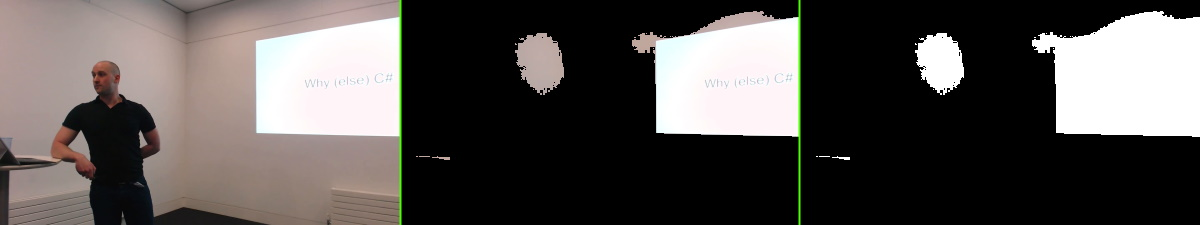Now we need to identify the largest "object" within this mask - wherever bright pixels are adjacent to other bright pixels, they will be considered part of the same object and we would expect there to be several such objects within the mask that has been generated.

To do so, I'll be reusing some more code from "How are barcodes read?" -

``````private static IEnumerable<IEnumerable<Point>> GetDistinctObjects(DataRectangle<bool> mask)
{
// Flood fill areas in the mask to create distinct areas
var allPoints = mask
.Enumerate()
while (allPoints.Any())
{
var currentPoint = allPoints.First();
var pointsInObject = GetPointsInObject(currentPoint).ToArray();
foreach (var point in pointsInObject)
allPoints.Remove(point);
yield return pointsInObject;
}

// Inspired by code at
// https://simpledevcode.wordpress.com/2015/12/29/flood-fill-algorithm-using-c-net/
IEnumerable<Point> GetPointsInObject(Point startAt)
{
var pixels = new Stack<Point>();
pixels.Push(startAt);

var valueAtOriginPoint = mask[startAt.X, startAt.Y];
var filledPixels = new HashSet<Point>();
while (pixels.Count > 0)
{
var currentPoint = pixels.Pop();
if ((currentPoint.X < 0) || (currentPoint.X >= mask.Width)
|| (currentPoint.Y < 0) || (currentPoint.Y >= mask.Height))
continue;

if ((mask[currentPoint.X, currentPoint.Y] == valueAtOriginPoint)
&& !filledPixels.Contains(currentPoint))
{
pixels.Push(new Point(currentPoint.X - 1, currentPoint.Y));
pixels.Push(new Point(currentPoint.X + 1, currentPoint.Y));
pixels.Push(new Point(currentPoint.X, currentPoint.Y - 1));
pixels.Push(new Point(currentPoint.X, currentPoint.Y + 1));
}
}
return filledPixels;
}
}
``````

As the code mentions, this is based on an article "Flood Fill algorithm (using C#.NET)" and its output is a list of objects, where each object is a list of points within that object. So the way to determine which object is largest is to take the one that contains the most points!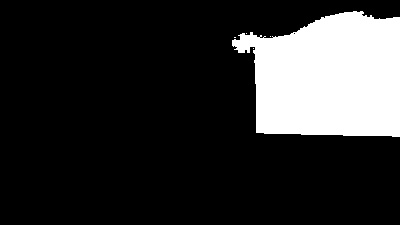``````var pointsInLargestHighlightedArea = GetDistinctObjects(mask)
.OrderByDescending(points => points.Count())
.FirstOrDefault();
``````

(Note: If `pointsInLargestHighlightedArea` is null then we need to escape out of the method that we're in because the source image didn't produce a mask with any highlighted objects - this could happen if the image has every single with the same colour, for example; an edge case, surely, but one that we should handle)

From this largest object, we want to find a bounding quadrilateral, which we do by looking at every point and finding the one closest to the top left of the image (because this will be the top left of the bounding area), the point closest to the top right of the image (for the top right of the bounding area) and the same for the points closest to the bottom left and bottom right.

This can be achieved by calculating, for each point in the object, the distances from each of the corners to the points and then determining which points have the shortest distances - eg.

``````var distancesOfPointsFromImageCorners = pointsInLargeHighlightedArea
.Select(p =>
{
// To work out distance from the top left, you would use Pythagoras to take the
// squared horizontal distance of the point from the left of the image and add
// that to the squared vertical distance of the point from the top of the image,
// then you would square root that sum. In this case, we only want to be able to
// compare determine which distances are smaller or larger and we don't actually
// care about the precise distances themselves and so we can save ourselves from
// performing that final square root calculation.
var distanceFromRight = greyScaleImageData.Width - p.X;
var distanceFromBottom = greyScaleImageData.Height - p.Y;
var fromLeftScore = p.X * p.X;
var fromTopScore = p.Y * p.Y;
var fromRightScore = distanceFromRight * distanceFromRight;
var fromBottomScore = distanceFromBottom * distanceFromBottom;
return new
{
Point = p,
FromTopLeft = fromLeftScore + fromTopScore,
FromTopRight = fromRightScore + fromTopScore,
FromBottomLeft = fromLeftScore + fromBottomScore,
FromBottomRight = fromRightScore + fromBottomScore
};
})
.ToArray(); // Call ToArray to avoid repeating this enumeration four times below

var topLeft = distancesOfPointsFromImageCorners.OrderBy(p => p.FromTopLeft).First().Point;
var topRight = distancesOfPointsFromImageCorners.OrderBy(p => p.FromTopRight).First().Point;
var bottomLeft = distancesOfPointsFromImageCorners.OrderBy(p => p.FromBottomLeft).First().Point;
var bottomRight = distancesOfPointsFromImageCorners.OrderBy(p => p.FromBottomRight).First().Point;
``````

Finally, because we want to find the bounding area of the largest object in the original image, we may need to multiply up the bounds that we just found because we shrank the image down if either dimension was larger than 400px and we were performing calculations on that smaller version.

We can tell how much we reduced the data by looking at the width of the original image and comparing it to the width of the greyScaleImageData `DataRectangle` that was generated from the shrunken version of the image:

``````var reducedImageSideBy = (double)image.Width / greyScaleImageData.Width;
``````

Now we only need a function that will multiply the bounding area that we've got according to this value, while ensuring that none of the point values are multiplied such that they exceed the bounds of the original image:

``````private static (Point TopLeft, Point TopRight, Point BottomRight, Point BottomLeft) Resize(
Point topLeft,
Point topRight,
Point bottomRight,
Point bottomLeft,
double resizeBy,
int minX,
int maxX,
int minY,
int maxY)
{
if (resizeBy <= 0)
throw new ArgumentOutOfRangeException("must be a positive value", nameof(resizeBy));

return (
Constrain(Multiply(topLeft)),
Constrain(Multiply(topRight)),
Constrain(Multiply(bottomRight)),
Constrain(Multiply(bottomLeft))
);

Point Multiply(Point p) =>
new Point((int)Math.Round(p.X * resizeBy), (int)Math.Round(p.Y * resizeBy));

Point Constrain(Point p) =>
new Point(Math.Min(Math.Max(p.X, minX), maxX), Math.Min(Math.Max(p.Y, minY), maxY));
}
``````

The final bounding area for the largest bright area of an image is now retrieved like this:

``````var bounds = Resize(
topLeft,
topRight,
bottomRight,
bottomLeft,
reducedImageSideBy,
minX: 0,
maxX: image.Width - 1,
minY: 0,
maxY: image.Height - 1
);
``````

For the example image that we're looking at, this area is outlined liked this: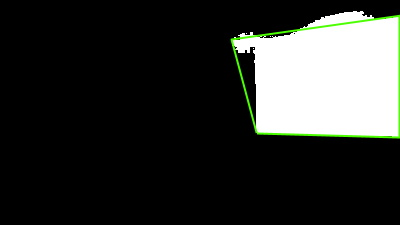### Applying the process to multiple images

Say that we put all of the above functionality into a method called `GetMostHighlightedArea` that took a `Bitmap` to process and returned a tuple of the four points that represented the bounds of the brightest area, we could then easily prepare a LINQ statement that ran that code and found the most common brightest-area-bounds across all of the source images that I have. (As I said before, the largest-bounded-area will vary from image to image in my example as the camera recording the session gained and lost focus)

``````var files = new DirectoryInfo("Frames").EnumerateFiles("*.jpg");
var (topLeft, topRight, bottomRight, bottomLeft) = files
.Select(file =>
{
using var image = new Bitmap(file.FullName);
return IlluminatedAreaLocator.GetMostHighlightedArea(image);
})
.GroupBy(area => area)
.OrderByDescending(group => group.Count())
.Select(group => group.Key)
.FirstOrDefault();
``````

Presuming that there is a folder called "Frames" in the output folder of project*, this will read them all, look for the largest bright area on each of them individually, then return the area that appears most often across all of the images. (Note: If there are no images to read then the `FirstOrDefault` call at the bottom will return a default tuple-of-four-Points, which will be 4x (0,0) values)

* (Since you probably don't happen to have a bunch of images from a video of my presentation lying around, see the next section for some code that will download some in case you want to try this all out!)

This ties in nicely with my recent post "Parallelising (LINQ) work in C#" because the processing required for each image is..

1. Completely independent from the processing of the other images (important for parallelising work)
2. Expensive enough that the overhead from splitting the work into multiple threads and then combining their results back together would be overshadowed by the work performed (which is also important for parallelising work - if individual tasks are too small and the computer spends more time scheduling the work on threads and then pulling all the results back together than it does on actually performing that work then using multiple threads can be slower than using a single one!)

All that we would have to change in order to use multiple threads to process multiple images is the addition of a single line:

``````var files = new DirectoryInfo("Frames").EnumerateFiles("*.jpg");
var (topLeft, topRight, bottomRight, bottomLeft) = files
.AsParallel() // <- WOO!! This is all that we needed to add!
.Select(file =>
{
using var image = new Bitmap(file.FullName);
return IlluminatedAreaLocator.GetMostHighlightedArea(image);
})
.GroupBy(area => area)
.OrderByDescending(group => group.Count())
.Select(group => group.Key)
.FirstOrDefault();
``````

(Parallelisation sidebar: When we split up the work like this, if the processing for each image was solely in memory then it would be a no-brainer that using more threads would make sense - however, the processing for each image involves LOADING the image from disk and THEN processing it in memory and if you had a spinning rust hard disk then you may fear that trying to ask it to read multiple files simultaneously would be slower than asking it to read them one at a time because its poor little read heads have to physically move around the plates.. it turns out that this is not necessarily the case and that you can find more information in this article that I found interesting; "Performance Impact of Parallel Disk Access")

### Testing the code on your own computer

I haven't quite finished yet but I figured that there may be some wild people out there that would like to try running this code locally themselves - maybe just to see it work or maybe even to get it working and then chop and change it for some new and exciting purpose!

To this end, I have some sample frames available from this video that I'm trying to fix - with varying levels of fuzziness present. To download them, use the following method:

``````private static async Task EnsureSamplesAvailable(DirectoryInfo framesfolder)
{
// Note: The GitHub API is rate limited quite severely for non-authenticated apps, so we just
// only call use it if the framesFolder doesn't exist or is empty - if there are already files
// in there then we presume that we downloaded them on a previous run (if the API is hit too
// often then it will return a 403 "rate limited" response)
if (framesfolder.Exists && framesfolder.EnumerateFiles().Any())
{
return;
}

if (!framesfolder.Exists)
framesfolder.Create();

string namesAndUrlsJson;
using (var client = new WebClient())
{
// The API refuses requests without a User Agent, so set one before calling (see
// https://docs.github.com/en/rest/overview/resources-in-the-rest-api#user-agent-required)
"https://api.github.com/repos/" +
"ProductiveRage/NaivePerspectiveCorrection/contents/Samples/Frames"
));
}

// Deserialise the response into an array of entries that have Name and Download_Url properties
var namesAndUrls = JsonConvert.DeserializeAnonymousType(
namesAndUrlsJson,
new[] { new { Name = "", Download_Url = (Uri?)null } }
);
if (namesAndUrls is null)
{
Console.WriteLine("GitHub reported zero sample images to download");
return;
}

.Select(async entry =>
{
using var client = new WebClient();
Path.Combine(framesfolder.FullName, entry.Name)
);
})
);

}
``````

.. and call it with the following argument, presuming you're trying to read images from the "Frames" folder as the code earlier illustrated:

``````await EnsureSamplesAvailable(new DirectoryInfo("Frames"));
``````

### Filtering out intro/outro slides

So I said earlier that it would also be nice if I could programmatically identify which frames were part of the intro/outro animations of the video that I'm looking at.

It feels logical that any frame that is of the actual presentation will have a fairly similarly-sized-and-located bright area (where a slide is being projected onto a wall in a darkened room) while any frame that is part of an intro/outro animation won't. So we should be able to take the most-common-largest-brightest-area and then look at every frame and see if its largest bright area is approximately the same - if it's similar enough then it's probably a frame that is part of the projection but if it's too dissimilar then it's probably not.

Rather than waste time going too far down a rabbit hole that I've found won't immediately result in success, I'm going to use a slightly altered version of that plan (I'll explain why in a moment). I'm still going to take that common largest brightest area and compare the largest bright area on each frame to it but, instead of saying "largest-bright-area-is-close-enough-to-the-most-common = presentation frame / largest-bright-area-not-close-enough = intro or outro", I'm going to find the first frame whose largest bright area is close enough and the last frame that is and declare that that range is probably where the frames for the presentation are.

The reason that I'm going to do this is that I found that there are some slides with more variance that can skew the results if the first approach was taken - if a frame in the middle of the presentation is so blurry that the range in intensity from darkest pixel to brightest pixel is squashed down too far then it can result in it identifying a largest bright area that isn't an accurate representation of the image. It's quite possible that I could still have made the first approach work by tweaking some other parameters in the image processing - such as considering changing that arbitrary "create a mask where the intensity threshold is 2/3 of the range of the brightness of all pixels" (maybe 3/4 would have worked better?), for example - but I know that this second approach works for my data and so I didn't pursue the first one too hard.

To do this, though, we are going to need to know what order the frames are supposed to appear in - it's no longer sufficient for there to simply be a list of images that are frames out of the video, we now need to know what were they appeared relative to each other. This is simple enough with my data because they all have names like "frame_1052.jpg" where 1052 is the frame index from the original video.

So I'm going to change the frame-image-loading code to look like this:

``````// Get all filenames, parse the frame index from them and discard any that don't
// match the filename pattern that is expected (eg. "frame_1052.jpg")
var frameIndexMatcher = new Regex(@"frame_(\d+)\.jpg", RegexOptions.IgnoreCase);
var files = new DirectoryInfo("Frames")
.EnumerateFiles()
.Select(file =>
{
var frameIndexMatch = frameIndexMatcher.Match(file.Name);
return frameIndexMatch.Success
? (file.FullName, FrameIndex: int.Parse(frameIndexMatch.Groups.Value))
: default;
})
.Where(entry => entry != default);

// Get the largest bright area for each file
var allFrameHighlightedAreas = files
.AsParallel()
.Select(file =>
{
using var image = new Bitmap(file.FullName);
return (
file.FrameIndex,
HighlightedArea: IlluminatedAreaLocator.GetMostHighlightedArea(image)
);
})
.ToArray()

// Get the most common largest bright area across all of the images
var (topLeft, topRight, bottomRight, bottomLeft) = allFrameHighlightedAreas
.GroupBy(entry => entry.HighlightedArea)
.OrderByDescending(group => group.Count())
.Select(group => group.Key)
.FirstOrDefault();
``````

(Note that I'm calling `ToArray()` when declaring `allFrameHighlightedAreas` - that's to store the results now because I know that I'm going to need every result in the list that is generated and because I'm going to enumerate it twice in the work outlined here, so there's no point leaving `allFrameHighlightedAreas` to be a lazily-evaluated `IEnumerable` that would be recalculated each time it was looped over; then it would be doing all of the `IlluminatedAreaLocator.GetMostHighlightedArea` calculations for each image twice if enumerated the list twice, which would just be wasteful!)

Now to look at the `allFrameHighlightedAreas` list and try to decide if each `HighlightedArea` value is close enough to the most common area that we found. I'm going to use a very simple algorithm for this - I'm going to:

1. Take all four points from the `HighlightedArea` on each entry in `allFrameHighlightedAreas`
2. Take all four points from the most common area (which are the `topLeft`, `topRight`, `bottomRight`, `bottomLeft` values that we already have in the code above)
3. Take the differences in `X` value between all four points in these two areas and add them up
4. Compare this difference to the width of the most common highlighted area - if it's too big of a proportion (say if the sum of the `X` differences is greater than 20% of the width of the entire area) then we'll say it's not a match and drop out of this list
5. If the `X` values aren't too bad then we'll take the differences in `Y` value between all four points in these two areas and add those up
6. That total will be compared to the height of the most common highlighted area - if it's more than the 20% threshold then we'll say that it's not a match
7. If we got to here then we'll say that the highlighted area in the current frame is close enough to the most common highlighted area and so the current frame probably is part of the presentation - yay!

In code:

``````var highlightedAreaWidth = Math.Max(topRight.X, bottomRight.X) - Math.Min(topLeft.X, bottomLeft.X);
var highlightedAreaHeight = Math.Max(bottomLeft.Y, bottomRight.Y) - Math.Min(topLeft.Y, topRight.Y);
const double thresholdForPointVarianceComparedToAreaSize = 0.2;
var frameIndexesThatHaveTheMostCommonHighlightedArea = allFrameHighlightedAreas
.Where(entry =>
{
var (entryTL, entryTR, entryBR, entryBL) = entry.HighlightedArea;
var xVariance =
new[]
{
entryBL.X - bottomLeft.X,
entryBR.X - bottomRight.X,
entryTL.X - topLeft.X,
entryTR.X - topRight.X
}
.Sum(Math.Abs);
var yVariance =
new[]
{
entryBL.Y - bottomLeft.Y,
entryBR.Y - bottomRight.Y,
entryTL.Y - topLeft.Y,
entryTR.Y - topRight.Y
}
.Sum(Math.Abs);
return
(xVariance <= highlightedAreaWidth * thresholdForPointVarianceComparedToAreaSize) &&
(yVariance <= highlightedAreaHeight * thresholdForPointVarianceComparedToAreaSize);
})
.Select(entry => entry.FrameIndex)
.ToArray();
``````

This gives us a `frameIndexesThatHaveTheMostCommonHighlightedArea` array of frame indexes that have a largest brightest area that is fairly close to the most common one. So to decide which frames are probably the start of the presentation and the end, we simply need to say:

``````var firstFrameIndex = frameIndexesThatHaveTheMostCommonHighlightedArea.Min();
var lasttFrameIndex = frameIndexesThatHaveTheMostCommonHighlightedArea.Max();
``````

Any frames whose index is less than `firstFrameIndex` or greater than `lastFrameIndex` is probably part of the intro or outro sequence - eg.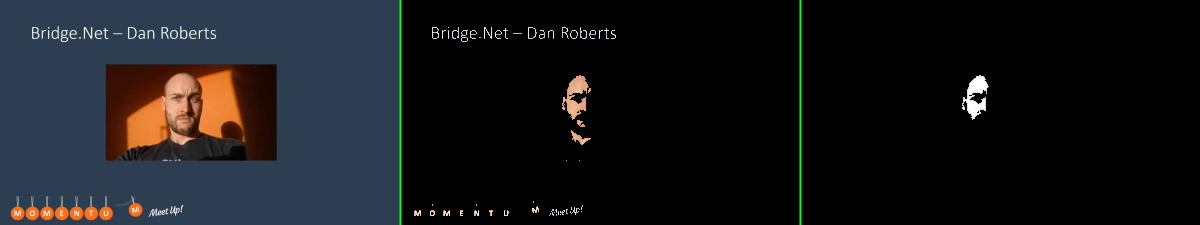Any frames whose index is within the `firstFrameIndex` / `lastFrameIndex` range is probably part of the presentation - eg.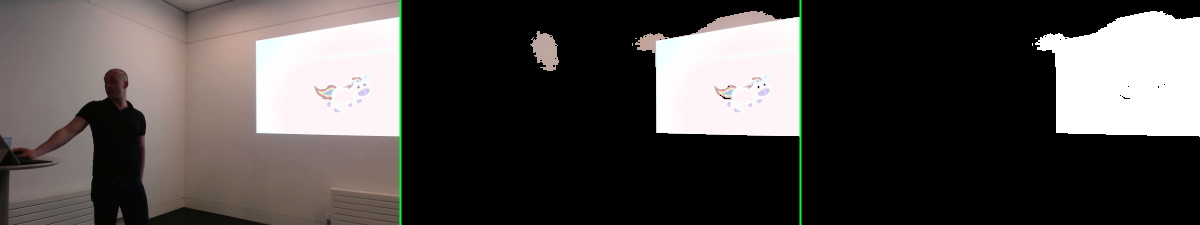### Coming soon

As the title of this post strongly suggests, this is only the first step in my desire to fix up my blurry presentation video. What I'm going to have to cover in the future is to:

1. Extract the content from the most-common-brightest-area in each frame of the video that is part of the presentation and contort it back into a rectangle - undoing the distortion that is introduced by perspective due to the position of the camera and where the slides were projected in the room (I'll be tackling this in a slightly approximate-but-good-enough manner because to do it super accurately requires lots of complicated maths and I've managed to forget nearly all of the maths degree that I got twenty years ago!)
2. Find a way to compare the perspective-corrected projections from each frame against a clean image of the original slide deck and work out which slide each frame is most similar to (this should be possible with some surprisingly rudimentary calculations inspired by some of the image preprocessing that I've mentioned in a couple of my posts that touch on machine learning but without requiring any machine learning itself)
3. Some tweaks that were required to get the best results with my particular images (for example, when I described the `GetMostHighlightedArea` function earlier, I picked 400px as an arbitrary value to resize images to before greyscaling them, masking them and looking for their largest bright area; maybe it will turn out that smaller or larger values for that process result in improved or worsened results - we'll find out!)

Once this is all done, I will take the original frame images and, for each one, overlay a clean version of the slide that appeared blurrily in the frame (again, I'll have clean versions of each slide from the original slide deck that I produced, so that should be an easy part) - then I'll mash them all back together into a new video, combined with the original audio. To do this (the video work), I'll likely use the same tool that I used to extract the individual frame files from the video in the first place - the famous FFmpeg!

I doubt that I'll have a post on this last section as it would only be a small amount of C# code that combines two images for each frame, writes the results to disk, followed by me making a command line call to FFmpeg to produce the video - and I don't think that there's anything particularly exciting there! If I get this all completed, though, I will - of course - link to the fixed-up presentation video.. because why not shameless plug myself given any opportunity!

Posted at 21:06